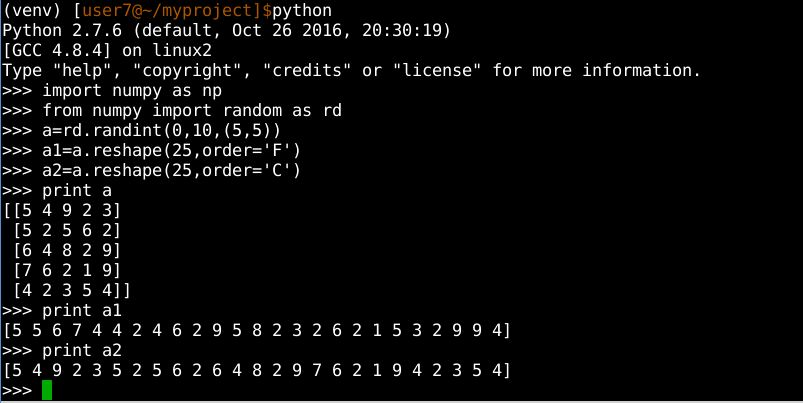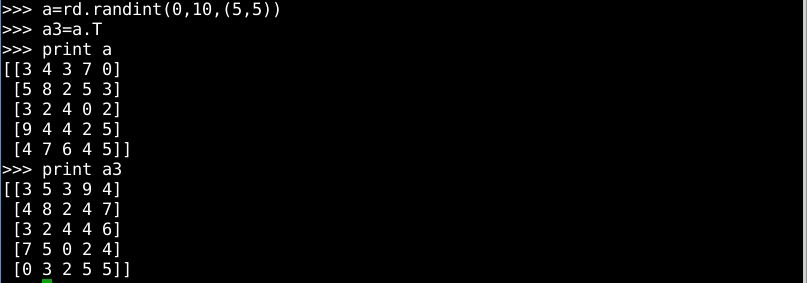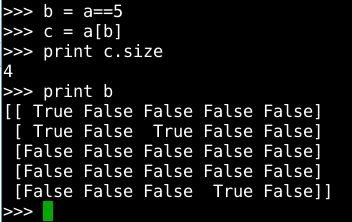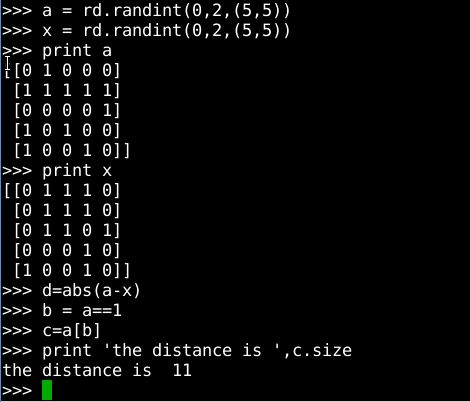• 工作中经常遇到求矩阵中非零元素个数的个数（L0范式） 下面介绍几种方法： 1、常规思路 import time import numpy as np data = np.array([ [5.0, 3.0, 4.0, 4.0, 0.0], [3.0, 1.0, 2.0, 3.0, 3.0], [4.0, 3.0, ...
工作中经常遇到求矩阵中非零元素个数的个数（L0范式） 下面介绍几种方法：
1、常规思路
import time
import numpy as np
data = np.array([
[5.0, 3.0, 4.0, 4.0, 0.0],
[3.0, 1.0, 2.0, 3.0, 3.0],
[4.0, 3.0, 4.0, 3.0, 5.0],
[3.0, 3.0, 1.0, 5.0, 4.0],
[1.0, 5.0, 5.0, 2.0, 1.0]
])
x,y = data.shape
num = 0
for i in range(0, x):
for j in range(0, y):
if array[i][j] > 0:
num += 1
print("L0 of data is:", num)

这是大多数人多常规思路，但是该方法在小矩阵，或小数据集上没有问题，一旦遇到大矩阵或者大数据集，性能太低，耗时太长
2 、尝试使用np 内部方法
import time
import numpy as np
data = np.array([
[5.0, 3.0, 4.0, 4.0, 0.0],
[3.0, 1.0, 2.0, 3.0, 3.0],
[4.0, 3.0, 4.0, 3.0, 5.0],
[3.0, 3.0, 1.0, 5.0, 4.0],
[1.0, 5.0, 5.0, 2.0, 1.0]
])
data[data < 0 ] = 0
data[data > 0] = 1
num = array.sum()
print("L0 of data is:", num)

这种方式就可以大大提高处理性能，面对大数据集也可以游刃有余
3、思路扩展 如果目标不是非零元素个数，而是小于或大于某一值threshold的元素个数呢
data[data < threshold ] = 0
data[data > threshold ] = 1
num = array.sum()
print("num of data is:", num)

展开全文python
• ## np的矩阵乘法

千次阅读 2019-03-21 20:55:52
np.dot（）方法 —— 矩阵乘法 Usage：np.dot( matrixA, matrixB ) or matrix.dot(matrixB) example： import numpy as np # define two matrixs here one = np.array([[1,2,3]]) two = np.array([,,]) #...
 np.dot（）方法 —— 矩阵乘法 Usage：np.dot( matrixA, matrixB ) or matrix.dot(matrixB) example： import numpy as np

# define two matrixs here
one = np.array([[1,2,3]])
two = np.array([,,])

# let's take a look at the shape of two matrixs
print(one.shape) # (1, 3)
print(two.shape) # (3,1)

print(np.dot(one,two)) # array()

print(one.dot(two))  # array()
  np.multiply () 或者 * ，矩阵内逐个元素相乘 element-wise example import numpy as np

# define two matrixs
one = np.array([[1,2,3],[4,5,6]])
two = np.array([[1,2,3],[4,5,6]])

print(np.multiply(one,two)

print( one * two )
 outputs: array([[ 1,  4,  9],
[16, 25, 36]])


另外，对于一维矩阵（相当于向量）来说，dot方法计算两者的内积 所谓内积，即对两个向量执行点乘运算，就是对这两个向量对应位一一相乘之后求和的操作，所得结果是一个标量。
import numpy as np

# define two vectors or one-dimention matrix
one = np.array([1,2,3])
two = np.array([3,4,5])

print(np.dot(one, two))   # 26


展开全文点乘
• np.repeat：复制的是多维数组的每一个元素；axis来控制复制的行和列 np.tile：复制的是多维数组本身； import numpy as np 通过help 查看基本的参数 help(np.repeat) help(np.tile) 案例对...
python 对矩阵进行复制操作 np.repeat 与 np.tile区别
####二者区别 二者执行的是均是复制操作； np.repeat：复制的是多维数组的每一个元素；axis来控制复制的行和列 np.tile：复制的是多维数组本身； import numpy as np 通过help 查看基本的参数 help(np.repeat) help(np.tile) ####案例对比
np.repeat
x = np.arange(1, 5).reshape(2, 2)
print(x)
[[1 2]
[3 4]]
print(np.repeat(x, 2))
[1 1 2 2 3 3 4 4]

对数组中的每一个元素进行复制 除了待重复的数组之外，只有一个额外的参数时，高维数组也会 flatten 至一维
c = np.array([1,2,3,4])
print(np.tile(c,(4,2)))
[[1 2 3 4 1 2 3 4]
[1 2 3 4 1 2 3 4]
[1 2 3 4 1 2 3 4]
[1 2 3 4 1 2 3 4]]

当然将高维 flatten 至一维，并非经常使用的操作，也即更经常地我们在某一轴上进行复制，比如在行的方向上（axis=1），在列的方向上（axis=0）：
print(np.repeat(x, 3, axis=1))
[[1 1 1 2 2 2]
[3 3 3 4 4 4]]
print(np.repeat(x, 3, axis=0))
[[1 2]
[1 2]
[1 2]
[3 4]
[3 4]
[3 4]]

当然更为灵活地也可以在某一轴的方向上（axis=0/1），对不同的行/列复制不同的次数：
print(np.repeat(x, (2, 1), axis=0))
[[1 2]
[1 2]
[3 4]]
print(np.repeat(x, (2, 1), axis=1))
[[1 1 2]
[3 3 4]]

#####np.tile
python numpy 下的 np.tile有些类似于 matlab 中的 repmat函数。不需要 axis 关键字参数，仅通过第二个参数便可指定在各个轴上的复制倍数。
a = np.arange(3)
print(a)
[0 1 2]
print(np.tile(a, 2))
[0 1 2 0 1 2]
print(np.tile(a, (2, 2)))
[[0 1 2 0 1 2]
[0 1 2 0 1 2]]

第二个参数便可指定在各个轴上的复制倍数。
b = np.arange(1, 5).reshape(2, 2)
print(b)
[[1 2]
[3 4]]
print(np.tile(b, 2))
[[1 2 1 2]
[3 4 3 4]]
print(np.tile(b, (1, 2)))
[[1 2 1 2]
[3 4 3 4]]展开全文numpy
• Python：随机矩阵生成，reshape，转置，统计矩阵中某元素个数，计算矩阵间汉明距离 初学python，遇到了许多问题，在此记录，以便大家共同学习。 本文主要是python矩阵操作的一些基本问题，用到了包为numpy。 一...
Python：随机矩阵生成，reshape，转置，统计矩阵中某元素个数，计算矩阵间汉明距离
初学python，遇到了许多问题，在此记录，以便大家共同学习。  本文主要是python矩阵操作的一些基本问题，用到了包为numpy。
一、随机矩阵生成以及reshape、转置问题
python代码
[python]
import numpy as np
from numpy import random as rd
a = rd.randint(0,10,(5,5))  #生成一个尺寸为5*5，取值在0-10之间的随机矩阵
a1 = a.reshape(25,order='F')#25为reshape后的向量尺寸，order表示拉列向量的方式，‘F’表示按列拉
a2 = a.reshape(25,order='C')#‘C’表示按行，具体结果示例见下图
a3=a.T
print a
print a1
print a2图中a1和a2分别是将矩阵a按列与按行拉成的向量，从输出的结果可以看出’order‘不同参数对应的排列方法。图中a3为a的转置矩阵。
二、统计矩阵中某个元素的个数
python代码
[python]
b = a==3 #得到布尔矩阵b，a中等于5的地方为true，其他为false
z = a[b]
print z.size
print b
从运行结果，可以看出矩阵b的类型三、计算矩阵间汉明距离
汉明距离定义：汉明距离是使用在数据传输差错控制编码里面的，汉明距离是一个概念，它表示两个（相同长度）字对应位不同的数量，我们以d（x,y）表示两个字x,y之间的汉明距离。对两个字符串进行异或运算，并统计结果为1的个数，那么这个数就是汉明距离。出处：汉明距离百度百科  python 代码
[python]
from numpy import random as rd
a = rd.randint(0,2,(5,5))#随机生成两个0、1矩阵
x = rd.randint(0,2,(5,5))
print a
print x
d=abs(a-x) #两个矩阵求差，并取绝对值
b = d==1 #统计差矩阵中值为1的部分，即统计两个矩阵值不同的数为汉明距离
c=d[b]
print 'the distance is ',c.size
运行结果，这两个矩阵汉明距离为11申明
本文为本人原创，转载请注明出处！
参考文献
1、https://baike.baidu.com/item/%E6%B1%89%E6%98%8E%E8%B7%9D%E7%A6%BB/475174?fr=aladdin  2、  http://blog.csdn.net/Yan456jie/article/details/52096582
展开全文python numpy random 汉明距离
• ## np中的矩阵

万次阅读 2020-05-01 10:13:07
numpy中的ndarray方法和属性 ...比如说，二维数组相当于是一一维数组，而这一维数组中每个元素又是一一维数组。所以这一维数组就是NumPy中的轴（axes），而轴的数量——秩，就是数组的维。 Numpy库...
• 注意矩阵乘法运算中的“*”和“np.dot()”是不一样的。 “*”的意思是给定一大小为(4,3)的矩阵A和一大小为(4,3)的矩阵B，两者使用“A*B”得到的矩阵的...当使用“np.dot()”时，需要一个矩阵为(4,3)，另一矩...
• numpy 矩阵元素的拼接(concatenate) numpy.append(arr, values, axis=None) 官方文档 示例 Examples >>>np.append([1, 2, 3], [[4, 5, 6], [7, 8, 9]]) array([1, 2, 3, ..., 7, 8, 9]) When axis is ...numpy python
• import numpy as np T = np.full((6, 6),3) print(T)
• 把向量或矩阵中所有元素相乘 import numpy as np mat= np.array([[1, 2, 3], [1, 2, 3], [1, 2, 3]]) print(np.prod(mat)) 结果： 216 示例2. 指定 axis Axis or axes along which a product is performed. 示例...python numpy 机器学习 数据挖掘 深度学习
• R语言随机替换矩阵元素 问题：给定一布尔矩阵X∈Rm×nX∈Rm×nX\in R^{m\times n}，要求随机抽取0.05%的元素，将0换成1，将1换成0。 可以用sample来选随机数。生成线性索引向量index：XXX看成一1×mn1×mn1\...
• 把一13的矩阵拼接在33的矩阵下方 随机生成两个矩阵 import numpy as np a=np.random.randint(1,9,size=9).reshape((3,3)) print(a) b=np.random.randint(1,9,size=3) print(b) 结果 [[5 8 5] [4 8 6] [8 6 4]] ...
• 同线性代数中矩阵乘法的定义： np.dot()np.dot(A, B)：对于二维矩阵，计算真正意义上的矩阵乘积，同线性代数中矩阵乘法的定义。对于一维矩阵，计算两者的内积。见如下Python代码：import numpy as np# 2-D array: 2...python multiply
• 我们平常使用矩阵的时候，通常会发现矩阵元素不能够满足我们的需求，其中一种情况就是矩阵内的元素要么过大，要么过小，最好将矩阵元素限制在某个范围之内。 代码： import numpy as np b=np.arange(1,13)....python numpy 数据结构
• >>> import numpy as np >>> a = np.array([[2,2],[4,4],... mean = np.mean(a) # 将上面二维矩阵的每个元素相加除以元素个数（求平均数） >>> mean 4.0 >>> mean2 = np.mean(a,python numpy 机器学习 深度学习
• Numpy中的矩阵向量乘法分别是np.dot(a,b)、np.multiply(a,b) 以及*，刚开始接触的时候比较模糊，于是自己整理了一下。先来介绍理论，然后再结合例子深入了解一下。 数组 矩阵 元素乘法 np.multiply(a,b) 或...numpy
• 描述：依次输出矩阵中最近邻的元素 例如：输入: [[1, 2, 3] [4, 5, 6] [7, 8, 9]] 输出： [[ 1. 2. 4. 5. 3. 6. 7. 8. 9.][ 2. 1. 3. 4. 5. 6. 7. 8. 9.][ 3. 2. 5. 6. 1. 4. 7. 8. 9.][ 4. 1. 2. ...
• python for循环随机矩阵中的每一个元素 from numpy import*; #不用像as np 那样每次调用时输入np X=random.random((0,2)size(4,4))#创建一只有0,1分布的4×4的随机矩阵 rows,cols=X.shape#读取X的行列 for i in...
• >... a = np.random.random((3,4)) >>> a array([[0.890582 , 0.43671675, 0.19531267, 0.13555252], [0.17254557, 0.3088476 , 0.36916878, 0.81551741], [0.31869139, 0.1616848...
• 昨天在搭建感知机模型的时候，遇到了一维矩阵中数据提取不出来的情况，所以今天复习一下一维矩阵中数据的提取...在numpy中，使用np.matrix()可以将numpy数据转换为矩阵，mat()的具体操作方法可以参考这篇博客numpy之pytpython numpy
• 这时候就需要原矩阵SSS中不为0元素和为0元素的索引值，这方法在matlab中是find方法，在用python实现时就需要np.nonzero()。下面看一段代码： import numpy as np a = np.arange(12).reshape(3, 4) print(a) [[ 0 ...numpy python
• python中numpy包的array矩阵，判断某个元素的个数 import numpy as np a=np.array([[ 2, 7, 4, 2], [35, 9, 1, 5], [22, 12, 3, 2]]) print(np.sum(a==2)) ## 2的个数 输出为 3python numpy array
• 矩阵乘法np.dot()及np.multipy()区别 1. 线性代数中矩阵乘法: np.dot() import numpy as np ​ # 2 x 3 matrix1 = np.array([[1, 2, 3], [4, 5, 6]]) ​ # 3 x 2 matrix2 = ...
• import numpy as np &gt;&gt;&gt; t=np.arange(4) # 插入值0-3 &gt;&gt;&gt; t array([0, 1, 2, 3]) &gt;&gt;&gt; t.transpose() array([0, 1, 2, 3]) &gt;&gt;&...
• 让我们看另一例子，其中矩阵元素不是整数的平方。 这次，我们将使用Python解释器。 >>> import numpy >>> >>> array = numpy.array([[1, 3], [5, 7]], dtype=numpy.float) >>> >>> print(array) [[1. 3.] [5. 7.]...
• data数组中小于1的元素都替换为...统计元素个数： import numpy as np from collections import Counter a = np.array([[1,2],[3,4]]) Counter(a.flatten()) # 二维数组需展平统计 Out: Counter({1: 1, 2: 1, 3: ...
• ## python-统计元素个数

万次阅读 2018-07-12 18:02:18
1. 统计数组中所有元素出现的次数&gt;&gt;&gt; y = np.array([1, 1, 2, 3, 3, 3]) &gt;&gt;&gt; key = np.unique(y) &gt;&gt;&gt; result = {} &gt;&gt;&gt; for k...
• import numpy as np arr = np.random.random((2,3)) # 需要进行统计的数据 arr_gb = arr.flatten() # 数组转为1维 arr_gb = pd.Series(arr_gb) # 转换数据类型 arr_gb = arr_gb.value_counts()...python...